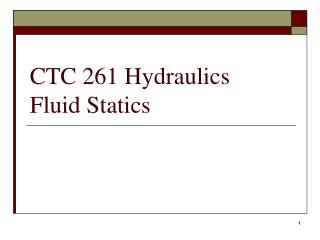Download PresentationCTC 261 Hydraulics Fluid Statics

# CTC 261 Hydraulics Fluid Statics - PowerPoint PPT PresentationDownload Presentation## CTC 261 Hydraulics Fluid Statics

- - - - - - - - - - - - - - - - - - - - - - - - - - - E N D - - - - - - - - - - - - - - - - - - - - - - - - - - -
##### Presentation Transcript

1. CTC 261 HydraulicsFluid Statics

2. Objectives • Know the difference between absolute and gage pressure • Know how to calculate hydrostatic pressures and forces on a horizontal plane surface • Know how to convert between pressure and pressure head • Know how to solve manometer problems • Know how to calculate hydrostatic pressures and forces on a vertical plane surface

3. Absolute Pressure Absolute pressure is measured in reference to a vacuum or zero pressure. Pressure at sea level is 1 atmosphere, 101.3 kPa, or 14.70 psi. Pressures measured by an absolute pressure transducer are always positive because these devices are referenced to a perfect vacuum in which absolute pressure is zero. Zero referencing is accomplished by completely evacuating and sealing the interior or reference side of the transducer. REF: http://pubs.usgs.gov/twri/twri8a3/#N1017F

4. Gage Pressure Gage pressure is measured in reference to atmospheric pressure at mean sea level. Pressures measured by a gage-pressure transducer are positive for pressures greater than sea-level pressure and negative for pressure less than sea-level pressure. Thus, atmospheric pressure measurements above sea-level datum are negative because atmospheric pressure decreases with altitude. Sea-level referencing is accomplished by sealing the interior or reference side of the transducer to atmospheric pressure at sea level. The term "gage pressure" is sometimes used to describe pressure measurements referenced to ambient atmospheric pressure other than to sea level. REF: http://pubs.usgs.gov/twri/twri8a3/#N1017F

5. Pressure • Vacuum pressure is the difference between atmospheric pressure and absolute pressure (vacuum pressure is always below atmospheric pressure) • Absolute pressure = Gage Pressure + Atmospheric Pressure • In civil engineering, gage pressure is what’s normally used (but specify in your units, psig)

6. Hydrostatic Pressure • Pressure which a fluid exerts on an object or container wall • Always acts through the center of pressure and is normal to the exposed surface • Hydrostatic pressure varies linearly with depth and is a function of depth and specific weight only

7. Hydrostatic Pressure and Resultant Force on a Horizontal Plane Surface • Pressure is uniform because the depth of liquid is the same across the plane surface • Pressure=Specific weight * depth (height)

8. Hydrostatic Pressure on a Horizontal Plane Surface Cylinder of water 1 foot high; area = 1 ft2 What is the pressure at the bottom of the cylinder? P=Specific Wt * Height of Water P=62.4 #/ ft2 F=Pressure * Area F=62.4 # 8

9. Force on Horizontal Plane Surface The magnitude of the force is 62.4# Where does it act? 9

10. Calculating Resultant Force • Magnitude of the force = pressure * area • Location of the force is at the centroid of the horizontal plane surface • Centroids of some simple shapes can be found in Angel

11. Pressure and Pressure Head • In civil engineering water pressure (psi) is often expressed in terms of water head (ft)

12. Converting Pressure to Pressure Head • Pressure Head = Pressure/Specific Weight • Example: • Atmospheric pressure = 14.7 psia • (14.7 psia)(144 in2/ft2)/(62.4#/ft3)=33.9 ft H2O The specific weight of Hg is 13.6 therefore the pressure head in terms of Hg would be 2.49 ft

13. Water is Heavy!!! 13 Meat Man http://www.youtube.com/watch?v=gmafAiLHUXI Meat Man @ 300’ of water depth (approx.135 psi) P=64.1 #/ft3 * 300 ft*/144 in2 per ft2 = 133.5 psi http://www.youtube.com/watch?v=LEY3fN4N3D8&NR=1

14. Manometer Principles Point 1: Pressure=0 Point 2: Pressure=h*specific weight of liquid Point 3: Pressure=Pressure @ 2 Point 4: Pressure=Pressure @ 3 –d*specific weight of liquid in vessel 4 d • As you go down pressure increases. • As you go up pressure decreases. • As long as you know pressure at some point (& fluid properties) you can determine pressures at all other points. http://www.energymanagertraining.com/energy_audit_instruments/manometers/principal%20of%20manometer.htm

15. Conundrum-----

16. Break

17. Hydrostatic Pressure on a Vertical, Rectangular Plane Surface • Pressure varies since height of fluid varies • Pressure distribution is linear • Resultant force = average pressure * surface area

18. Vertical, rectangular plane surface Consider a dam section 1’ wide and 10’ deep What is the pressure distribution and resultant force of the water pressure? Pressure @ top = 0 Pressure @ bottom = 624 #/ft2 Average Pressure = 312 #/ ft2 18

19. Vertical Rectangular Plane Surface Magnitude of force = Avg Pressure * Area F= 312 #/ ft2 * (10’x1’) =3,120# Where is the resultant force located? Centroid of the dam (10’by 1’)? Centroid of the pressure distribution (triangular)? 19

20. ANSWER Because the pressure varies with depth the resultant force does not acts at the centroid of the surface (rectangle), but acts at the centroid of the pressure distribution (triangular)

21. Example (1/2) Assume that freshly poured concrete exerts a hydrostatic force similar to that exerted by a liquid of equal specific weight What is the force acting on one side of a form that is 8’V by 4’H used for pouring a basement wall 21

22. Example (2/2) Assume concrete specific wt = 150#/ft3 Area = 8’x4’=32 ft2 Avg Pressure =(0*150+8*150)/2=600#/ft2 F=Avg Pressure*Area=600*32 F=19,200# = 9.6 tons Acting 2/3*8’=5.33’ from top 22

23. Submerged Vertical Rect. Plane Surface • Is the pressure distribution still a triangle?

24. Answer • For a submerged vertical rectangular plane surface the pressure distribution is a trapezoid (since the height is not zero at the top of the surface)

25. Next Lecture • Fluid Statics on an inclined, submerged surface • Buoyancy